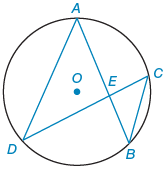Alyssa Z.

# Given:AE = 7, EB = 4 DC = 16. Find:DE and EC (Assume DE > EC.)

Consider the following theorem.

If two chords intersect within a circle, then the product of the lengths of the segments (parts) of one chord is equal to the product of the lengths of the segments of the other chord.

O is the center of the circle.A circle contains six labeled points and four line segments.

1. The center of the circle is point O.
2. Points ABC and D are on the circle. Point A is on the top middle, point B is on the bottom right, point C is slightly above the middle right, and point D is on the bottom left.
3. A line segment connects points A and B.
4. A line segment connects points C and D.
5. A line segment connects points A and D.
6. A line segment connects points C and B.
7. Point E is the intersection of line segments A B and C D. Point E is to the right and slightly below point O.
 Given:AE = 7 EB = 4 DC = 16 Find:DE and EC (Assume DE > EC.)Mark M.

What does the theorem state? Do you have difficulty with the English or with the Geometry?
Report

24d

By:Tutor
5 (20)

Math Tutor with Experience

## Still looking for help? Get the right answer, fast.

Get a free answer to a quick problem.
Most questions answered within 4 hours.

#### OR

Choose an expert and meet online. No packages or subscriptions, pay only for the time you need.